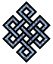fail2ban bad ip database: ip 178.91.253.19

| ip database | live view | stats | report | help | api key:

 ip: 178.91.253.19 hostname: 178.91.253.19 country:[KZ] Kazakhstan first reported: 18.09.2018 21:58.34 GMT+0200 last reported: 04.02.2019 05:34.34 GMT+0200 time period: 138d 08h 36m total reports: 10 reported by: 4 host(s) filter(s): ssh (7) ssh (3) tor exit node no badips.com db Lookupport scan of '178.91.253.19':

[-hide]
```# Nmap 6.40 scan initiated Tue Sep 18 21:59:02 2018 as: /usr/bin/nmap -sU -sS -O 178.91.253.19
Nmap scan report for 178.91.253.19
Host is up (0.093s latency).
Not shown: 1000 open|filtered ports, 976 filtered ports
PORT     STATE  SERVICE
21/tcp   closed ftp
22/tcp   open   ssh
25/tcp   closed smtp
80/tcp   open   http
443/tcp  open   https
993/tcp  closed imaps
3000/tcp closed ppp
3001/tcp closed nessus
3003/tcp closed cgms
3007/tcp closed lotusmtap
5432/tcp open   postgresql
8080/tcp open   http-proxy
8081/tcp open   blackice-icecap
8083/tcp closed us-srv
8084/tcp closed unknown
8085/tcp closed unknown
8086/tcp closed d-s-n
8087/tcp closed simplifymedia
8089/tcp closed unknown
8090/tcp open   unknown
No exact OS matches for host (If you know what OS is running on it, see http://nmap.org/submit/ ).
TCP/IP fingerprint:
OS:SCAN(V=6.40%E=4%D=9/18%OT=22%CT=21%CU=%PV=N%G=Y%TM=5BA15EFC%P=x86_64-pc-
OS:linux-gnu)SEQ(SP=107%GCD=1%ISR=10B%TI=Z%TS=8)OPS(O1=M5B4ST11NW7%O2=M5B4S
OS:T11NW7%O3=M5B4NNT11NW7%O4=M5B4ST11NW7%O5=M5B4ST11NW7%O6=M5B4ST11)WIN(W1=
OS:7120%W2=7120%W3=7120%W4=7120%W5=7120%W6=7120)ECN(R=Y%DF=Y%TG=40%W=7210%O
OS:=M5B4NNSNW7%CC=Y%Q=)T1(R=Y%DF=Y%TG=40%S=O%A=S+%F=AS%RD=0%Q=)T2(R=N)T3(R=
OS:N)T4(R=N)T5(R=Y%DF=Y%TG=40%W=0%S=Z%A=S+%F=AR%O=%RD=0%Q=)T6(R=N)T7(R=N)U1
OS:(R=N)IE(R=N)

OS detection performed. Please report any incorrect results at http://nmap.org/submit/ .
# Nmap done at Tue Sep 18 22:24:28 2018 -- 1 IP address (1 host up) scanned in 1527.77 seconds
```
```Σ = 64 | Δt = 0.0084271430969238s
```